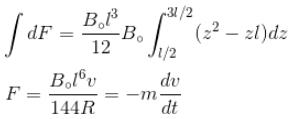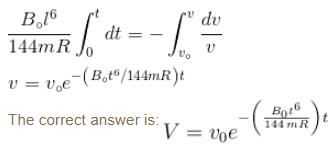Courses

# Faraday's Law MCQ Level - 2

## 10 Questions MCQ Test Topic wise Tests for IIT JAM Physics | Faraday's Law MCQ Level - 2

Description
This mock test of Faraday's Law MCQ Level - 2 for Physics helps you for every Physics entrance exam. This contains 10 Multiple Choice Questions for Physics Faraday's Law MCQ Level - 2 (mcq) to study with solutions a complete question bank. The solved questions answers in this Faraday's Law MCQ Level - 2 quiz give you a good mix of easy questions and tough questions. Physics students definitely take this Faraday's Law MCQ Level - 2 exercise for a better result in the exam. You can find other Faraday's Law MCQ Level - 2 extra questions, long questions & short questions for Physics on EduRev as well by searching above.
QUESTION: 1

### The current in inner coil is i = 2t2, as shown in figure. The current induced in the outer coil has a resistance of R.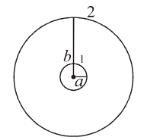Solution:

Let I  be the current be in the outer coil 2.
The field at centre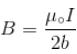The flux through the inner coil due to current in the outer coil 2,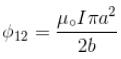So, Mutual inductance of outer coil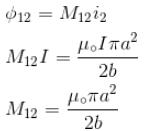Flux across the outer coil,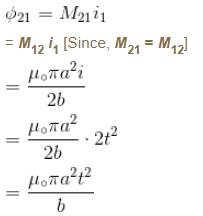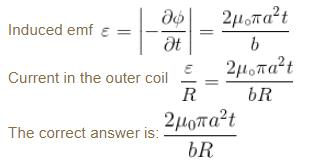QUESTION: 2

### A circular loop of radius a is made of a single turn of thin conducting wire. The self inductance of this loop is L. If the number of turns in the loop is increased from 1 to 8, the self inductance would be :

Solution:

Let us consider there are n  turns of a coil having radius a and current  I. Then magnetic field at the centre of the coil is given by.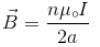Flux of magnetic field through a coil.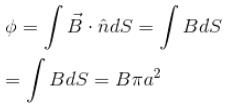Flux through n  coil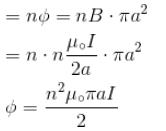Self inductance given by relation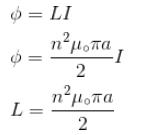If number of turns in the loop is increased from 1 to 8 then, Self inductance increased by 64 time.

QUESTION: 3

### A large circular coil of N  turns and radius R carries a time varying current I = I0 sin (ωt). A small circular coil of n  turns and radius r (r << R) is placed at the center of the large coil such that the coils are concentric and coplanar. The induced emf in the small coil :

Solution:

Flux through small coil is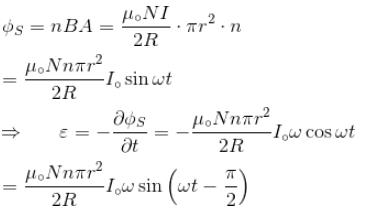Hence, emf  is lay behind the large coil current by π/2
The correct answer is: lags the current in the large coil by π/2

QUESTION: 4

A metal disc of radius a  rotates with an angular velocity ω about an axis perpendicular to its plane passing through its centre in a magnetic field of induction B  acting perpendicular to the plane of the disc. The induced emf between the rim and axis of the disc.

Solution:

In the presence of uniform magnetic field B perpendicular to the disc in outward direction (say) Force on a unit positive charge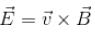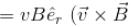will lie in radially outward direction)
Work done in moving a unit positive charge from centre to the rim is equal to potential difference ε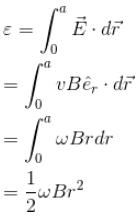The correct answer is: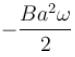QUESTION: 5

A wire coil of length l, mass m, is sliding without friction on conducting rail ax and by as shown in figure. The vertical rails are connected to one another via an external resistance  R. The entire circuit is placed in a region of space having a uniform magnetic field B. The field is perpendicular to the plane of circuit and directed outwards. The steady speed of rod cd  is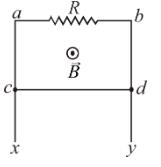Solution:

Let wire cd  is at distance x  from the edge be considered a plane surface bounded by the loop acdb  and unit normal vector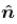lying in same direction as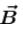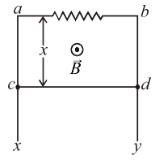Flux through area  acdb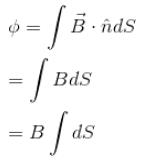Blv
Since, Flux is increasing with time, emf will be induced so as to circulate current resulting in weakening of magnetic field. So, current should flow from P  to  Q.
Current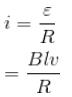Lorentz force acting on rod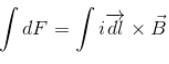ilB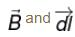are perpendicular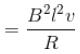(forward up the incline)

Free body diagram of slide is shown in figure.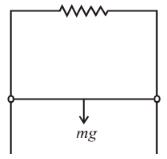There is no acceleration in direction perpendicular to plane of the loop.

So, N = O   [N  Normal reaction perpendicular to the plane]

But in the plane of the loop, the wire cd  slide down due to gravitational force acting downward and lorentz force acting upward.

So, In downward direction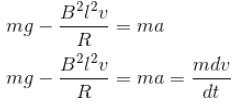But when we talk about steady speed,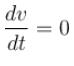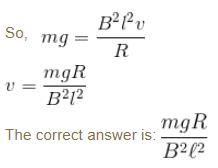QUESTION: 6

A loop is formed by two parallel conductor connected by a solenoid with inductance L and a conducting rod of mass m  which can freely (without friction) side over the conductors. The conductors are located in horizontal plane in a uniform vertical magnetic field B. The distance between the conductor is l. Then angular frequency of rod.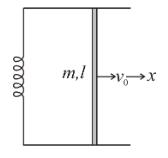Solution:

Let at any instant of time, velocity of the rod is  towards right. The current in the circuit is i. In the figure,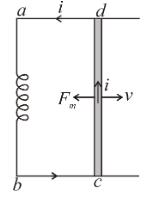Va – Vb = Vd – Vc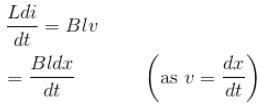i.e. Ldi = Bldx
Li
= Blx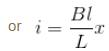Magnetic force on the rod at this instant is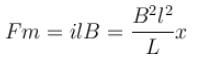Since, this force is in opposite direction of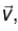so from Newton’s second law we an write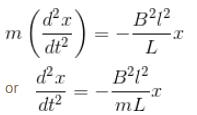Comparing this with equation of SHM, i.e.,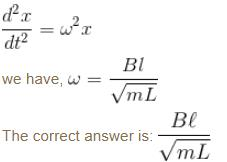QUESTION: 7

A conducting rod of length a is rotating with constant angular speed ω about its perpendicular bisector. A uniform magnetic field B exist parallel to the axis of rotation. The emf induced between two ends of the rod is.

Solution:

Let us calculate motional emf produced in the rod. Consider a unit positive charge on the rod at a distance r  from the centre. Let rod rotates with angular velocity ω. Velocity of this unit positive charge in magnetic field in transverse direction is ωr.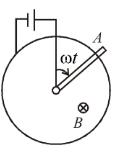Lorentz force acting on the unit charge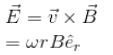Potential difference between A  and  O = VAO

work done in moving a unit positive charge from O  to A.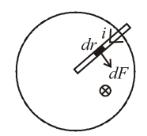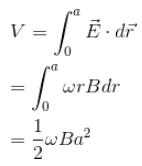The correct answer is: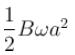QUESTION: 8

An infinite long wire carrying a current I(t) = I0 cos(ωt) is placed at a distance a from a square loop of side a  in the figure. If the resistance of the loop is  R, then the amplitude of the induced current in the loop is :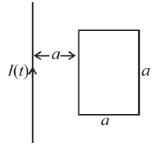Solution:

To find out the flux through the loop. Consider an element of length a and breadth dx at a distance x  from wire.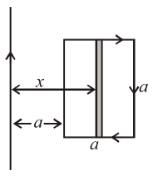∴  Area = adx = dS

Let the orientation of the loop is such that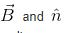lie in same direction,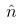is unit normal to surface.

Magnetic field due to long current carrying conductor wire.

at a distance x is given by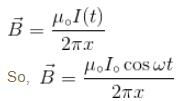Flux of magnetic field through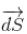element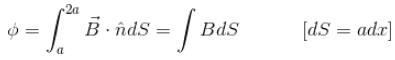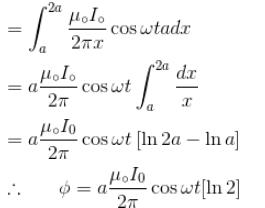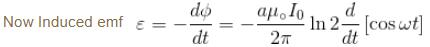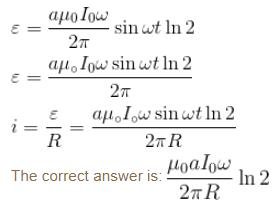QUESTION: 9

Two coil of self inductance L1 and L2 are placed closed to each other so that total flux in one coil is completely linked with other. If M is the mutual inductance between them, then :

Solution:

When the flux changes in the second coil due to change in the current in first coil, then the mutual inductance is given by the relation.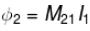Similarly, when flux changes in first coil due to change in the current in second coil.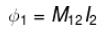From the Reciprocity Theorem
M21 = M12 = M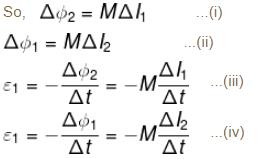From (iii) and (iv)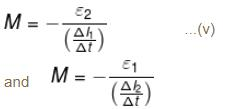Multiply (v) and (vi)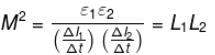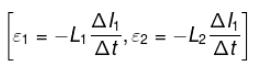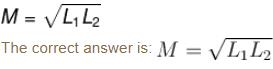QUESTION: 10

Two parallel rails separated by a distance l are connected through a resistance R  in yz plane. Magnetic field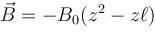is present in the direction perpendicular to the plane of the rails. A conducting rod of mass. m  placed on the rails and given a velocity of v0 parallel to the plane of rails. The expression for the velocity.

Solution:

Let the distance of slider from AB is y. Consider an element of length y and width dz  at a distance z from the y axis.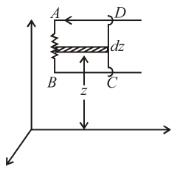dS = ydz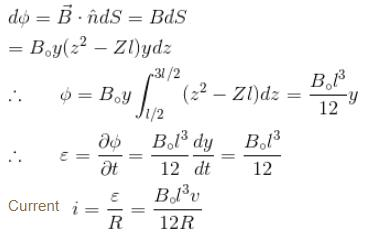consider a length dz of the rod

∴  Magnetic force on rod dF = iB·dz